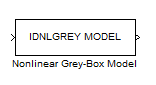Nonlinear Grey-Box Model

Simulate nonlinear grey-box model in Simulink software

Library

System Identification Toolbox

•Description

Simulates systems of nonlinear grey-box (idnlgrey) models.

Input

Input signal to the model.

Output

Output signal from the model.

Parameters

IDNLGREY model

Name of idnlgrey variable in the MATLAB® workspace.

Initial state

Specify the initial states as one of the following:

• 'z': Specifies zero, which corresponds to a system starting from rest.

• 'm': Specifies the internal initial states of the model.

• Vector of size equal to the number of states in the idnlgrey object.

• An initial state structure array. For information about creating this structure, type help idnlgrey/sim in the MATLAB Command Window.

Blocks

Introduced in R2008a

System Identification Toolbox DocumentationGet trial now